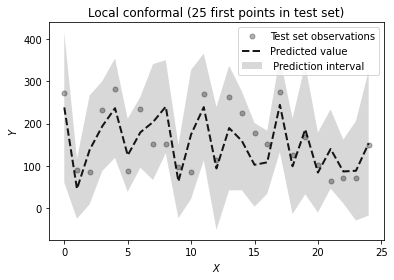This article was first published on T. Moudiki's Webpage - Python , and kindly contributed to python-bloggers. (You can report issue about the content on this page here)

In this post, I use the following Python packages:

• `BCN`: for adjusting Boosted Configuration Networks regression (see post 1 and post 2 for more
details on BCNs, and this notebook for classification examples) to `sklearn`’s `diabetes` dataset.

• `the-teller`: for interpreting BCNs, and obtaining prediction intervals. So far, as of october 2022, `the-teller` contains two methods for constructing prediction intervals: split conformal and local conformal. For more details on both of these methods, the interested reader can consult . Despite their potential drawbacks (listed in ), they’re model-agnostic, i.e they do not require in particular for the user to adjust a quantile regression model.

Content

• 0 – Install packages
• 1 – Import packages + load data
• 2 – Adjust BCN model to training set, evaluation on test set
• 3 – Adjust the teller’s Explainer to test set
• 4 – Prediction intervals on test set
• 4 – 1 Split conformal
• 4 – 2 Local conformal
• 5 – Plot prediction intervals

# 0 – Install packages

Package implementing Boosted Configuration Networks

```pip install BCN
```

Package for Machine Learning Explainability on tabular data

```pip install the-teller
```

Other packages

```pip install scikit-learn numpy
```

# 1 – Import packages + load data

```import BCN as bcn # takes a long time to run, ONLY the first time it's run
import teller as tr
import numpy as np
from sklearn.model_selection import train_test_split
from sklearn import metrics
from time import time
```

import `diabetes` dataset

```dataset = load_diabetes()
X = dataset.data
y = dataset.target

# split data into training test and test set
X_train, X_test, y_train, y_test = train_test_split(X, y,
test_size=0.2, random_state=13)
```

# 2 – Adjust BCN model to training set, evaluation on test set

```start = time()

regr_bcn = bcn.BCNRegressor(**{'B': 122,
'nu': 0.7862040612242153,
'col_sample': 0.6797268557618982,
'lam': 0.9244772287770061,
'r': 0.18915458607547728,
'tol': 9.670499926559012e-10})

regr_bcn.fit(X_train, y_train)

print(f"\nElapsed {time() - start}")

preds = regr_bcn.predict(X_test)

# Test set RMSE
print(np.sqrt(np.mean(np.square(y_test - preds))))
```
```Elapsed 0.641953706741333
54.50979209778048
```

# 3 – Adjust the teller’s Explainer to test set

```start = time()

# creating an Explainer for the fitted object `regr_bcn`
expr = tr.Explainer(obj=regr_bcn)

# confidence int. and tests on covariates' effects (Jackknife)
expr.fit(X_test, y_test, X_names=dataset.feature_names, method="ci")

# summary of results
expr.summary()

# timing
print(f"\n Elapsed: {time()-start}")
```
```Score (rmse):
54.51

Residuals:
Min         1Q   Median        3Q        Max
-119.79528 -30.293474 9.749065 45.169791 124.891462

Tests on marginal effects (Jackknife):
Estimate Std. Error  95% lbound  95% ubound  Pr(>|t|)
bmi  534.170652  10.538064  513.228463   555.11284       0.0  ***
s5   460.090298    11.7154  436.808403  483.372194       0.0  ***
bp   245.510926   7.073121  231.454584  259.567267       0.0  ***
s6   117.899033   8.865569  100.280578  135.517488       0.0  ***
s4     71.20425   4.885591   61.495165   80.913336       0.0  ***
age    3.144209   7.616405  -11.991795   18.280213  0.680742    -
s1    -31.76086   8.823018  -49.294754  -14.226967  0.000526  ***
s2  -104.139443   8.596589 -121.223357  -87.055529       0.0  ***
sex -167.461194    3.92965 -175.270546 -159.651841       0.0  ***
s3  -210.749954   5.580957 -221.840934 -199.658975       0.0  ***

Signif. codes:  0 ‘***’ 0.001 ‘**’ 0.01 ‘*’ 0.05 ‘.’ 0.1 ‘-’ 1

Multiple R-squared:  0.394,	Adjusted R-squared:  0.316

Elapsed: 0.1125936508178711
```

For more details on this output, you can read this post applying the-teller to xgboost’s explanation.

# 4 – 1 Split conformal

```pi = tr.PredictionInterval(regr_bcn, method="splitconformal", level=0.95)
pi.fit(X_train, y_train)
preds = pi.predict(X_test, return_pi=True)
```
```pred = preds
y_lower = preds
y_upper = preds
print(len(pred))

# compute and display the average coverage
print(np.mean((y_test >= y_lower) & (y_test <= y_upper)))

# prediction interval's length
length_pi = y_upper - y_lower
print(np.mean(length_pi))
```
```89
0.9438202247191011
209.55455918396666
```

# 4 – 2 Local conformal

```pi2 = tr.PredictionInterval(regr_bcn, method="localconformal", level=0.95)
pi2.fit(X_train, y_train)
preds2 = pi2.predict(X_test, return_pi=True)
```
```pred2 = preds2
y_lower2 = preds2
y_upper2 = preds2
print(len(pred2))

# compute and display the average coverage
print(np.mean((y_test >= y_lower2) & (y_test <= y_upper2)))

# prediction interval's length
length_pi2 = y_upper2 - y_lower2
print(np.mean(length_pi2))
```
```89
0.9550561797752809
229.03014949955275
```

# 5 – Plot prediction intervals

```import warnings
warnings.filterwarnings('ignore')

import matplotlib
matplotlib.use('Agg')
import matplotlib.pyplot as plt

import numpy as np
np.warnings.filterwarnings('ignore')

split_color = 'tomato'
local_color = 'gray'

%matplotlib inline
np.random.seed(1)

def plot_func(x,
y,
y_u=None,
y_l=None,
pred=None,
method_name="",
title=""):

fig = plt.figure()

plt.plot(x, y, 'k.', alpha=.3, markersize=10,
fillstyle='full', label=u'Test set observations')

if (y_u is not None) and (y_l is not None):
plt.fill(np.concatenate([x, x[::-1]]),
np.concatenate([y_u, y_l[::-1]]),
label = method_name + ' Prediction interval')

if pred is not None:
plt.plot(x, pred, 'k--', lw=2, alpha=0.9,
label=u'Predicted value')

#plt.ylim([-2.5, 7])
plt.xlabel('\$X\$')
plt.ylabel('\$Y\$')
plt.legend(loc='upper right')
plt.title(title)

plt.show()
```

Split conformal

```max_idx = 25
plot_func(x = range(max_idx),
y = y_test[0:max_idx],
y_u = y_upper[0:max_idx],
y_l = y_lower[0:max_idx],
pred = pred[0:max_idx],
title = f"Split conformal ({max_idx} first points in test set)")
```Local conformal

```max_idx = 25
plot_func(x = range(max_idx),
y = y_test[0:max_idx],
y_u = y_upper2[0:max_idx],
y_l = y_lower2[0:max_idx],
pred = pred2[0:max_idx],